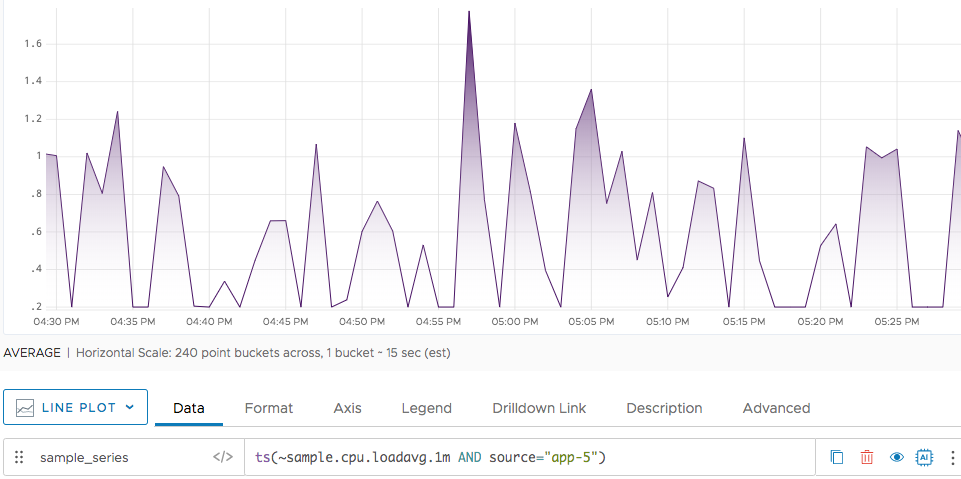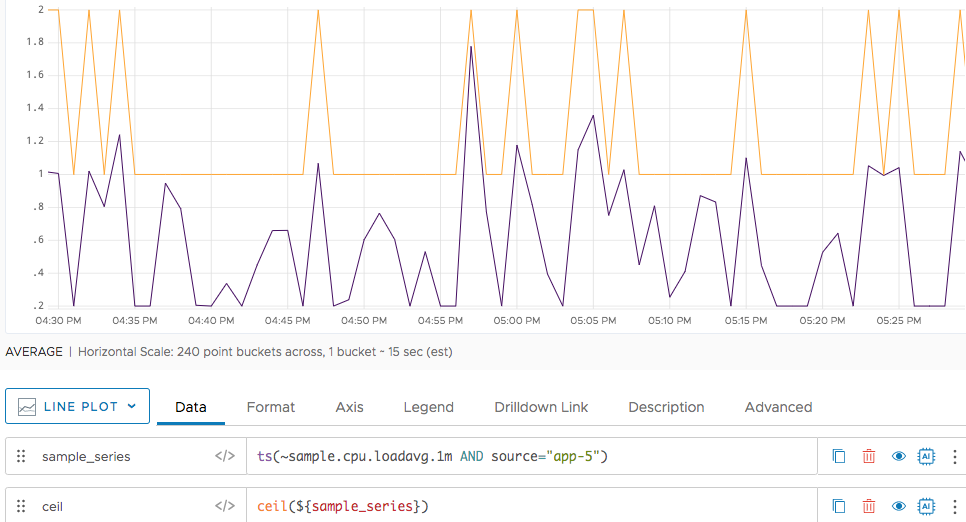Reference to the ceil() function

## Summary

``````ceil(<tsExpression>)
``````

Returns the ceiling for the time series described by the expression, by rounding any data values with decimals up to the nearest integer.

## Parameters

ParameterDescription
tsExpression Expression that describes the time series to return ceiling values for.

## Description

The `ceil()` function returns the ceiling for each data value in the time series described by the expression.

The ceiling of a data value is the smallest integer that is greater than or equal to that value:

Sample query with ceil()Sample resultInput data valueReturned value (ceiling)
`ceil(1.75)` 2 Positive, with fractional partNext higher integer
`ceil(-1.75)` -1 Negative, with fractional partInteger part of the input value
`ceil(1)` 1 Positive integerSame as the input value
`ceil(-1)` -1 Negative integerSame as the input value

`ceil()` returns a separate series of results for each time series described by the expression.

## Examples

Here’s a query that returns a time series that reports positive fractional values between .2 and 1.2. The color has been changed to dark purple.Now we apply `ceil()` to our original query. Notice that the values below 1 are mapped to 1, and the values from 1 to 1.2 are mapped to 2. The the ceil function uses orange.`floor()`
`round()`